# GMAT Data Sufficiency Logic: Tautological Statements

First, here are four DS practice problems:2) In the diagram above, the circle has its center at point O. Line BC touches the circle at point C, and the distance from B to C is 35 cm.  What is the area of the circle?

Statement #1: AB = 25 cm

Statement #2: angle OCB = 90°

3) At the 2016 convention for Aim Far Motivational Society (AFMS), each AFMS member had the option of inviting just one non-member guest.  Attending as the single guest of a member is the only way a non-member would be able to attend the convention.  At the 2016 convention, of the AFMS Convention Hall’s seats, 60% were occupied by AFMS members and 10% were occupied by non-member guest.  How many seat does the AFMS Convention Hall have?

Statement #1: If 60% of the members who didn’t bring a guest instead had brought one, then the hall would have been 100% full.

Statement #2:  The number of empty seats was half the number of the AFMS members present.## Tautologies

The purpose of language is to convey meaning.  For example, the following statements are true statement:

5) Saskatchewan is between Manitoba and Alberta.

6) The Yongle Emperor was the third emperor of the Ming Dynasty.

7) June 28, 1914 was a Sunday.

Each one of these sentences conveys specific information.  They are meaningful statements.  Of course, none of these are facts you need to have memorized for the GMAT.  Theoretically, any one of these could be a relevant sentence in some hypothetical GMAT RC passage.

By contrast, compare these sentences.

8) All pandas are pandas.

9) Every element on the Periodic Table is an element.

10) Each Tuesday in 2003 was between a Monday and a Wednesday.

Each one of these statements doesn’t really tell us anything.  Each one expresses something that is already 100% obvious before the statement was made.  Each statement contains no new information.  These are tautologies.  A tautology is a statement that contains absolutely no new information, because it simply restates what is already known.   In ordinary spoken language, a tautology sounds redundantly obvious: even though it is strictly true, it sounds like nonsense when it is spoken, because we have no idea what the motivation would be of a person speaking such a statement.   None of these could ever be a sentence in a GMAT RC passage, unless the passage were citing one of these as an example of a “tautology.”

## Mathematical Tautologies

There aren’t too many ways to disguise the same verbal information.  There are many more ways to disguise the same mathematical information. Consider the simple information a + b = c.   This could be rewritten as 2a + b = a + c or as –a = b – c.   This last equation, when divided by a, becomes: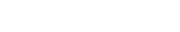This is starting to look very different, but all of these versions contain the same mathematical information.

That was a very simple algebraic example.  In other areas of math, the possibilities for redundant information multiply.   If you are told something is a rectangle, then it is redundant to add that one of the angles is a right angle.   If you are told the average of five numbers is 7, then it is redundant to add that their sum is 35.  If you are told that N is a prime number greater than 20, then it is redundant to add that N is odd.  If you are told that the probability of (P or Q) is less than 1/2, then it is redundant to add that the probability of P alone is less than 1/2.   The examples are endless.  If any redundant statement is added under the pretext of being an additional useful piece of information, such a statement is a tautology, a statement that adds no new useful information.

## Tautologies in GMAT Data Sufficiency

The basic setup of the GMAT DS is that the prompt provides partial or no information and then asks a question.  The prompt, by itself, is always insufficient.   The general is that each statement is supposed to provide a new piece of mathematical information, and the question is whether each statement, or their combination, provides information sufficient to answer the question.

Suppose one of the two statements is tautological: in other words, rather than add new independent information as statements usually do, it simply restates the information in the prompt or states something directly deducible from the prompt.   Of course, this statement adds nothing to the prompt: since the prompt is already insufficient, the tautological statement would have to be insufficient.  Furthermore, if the other statement, a genuine new piece of information, is also insufficient, combining it with the tautological statement will never produce sufficiency: in other words, one of the statements is tautological, (C) can never be the answer.

Notice that judging that a statement is tautological is a much more powerful logical conclusion that merely judging that the statement is insufficient.  If I decide that Statement #1 is insufficient, then, without looking at Statement #2, I know that the answer could be (B), (C), or (E).  By contrast, if I decide that Statement #1 is tautological, then, without looking at Statement #2, I know that the answer could be only (B) or (E).  If one statement is tautological, the entire DS problem reduces to a single binary choice about the other statement.

There are many different ways to rephrase mathematical information, so it can be tricky to recognize a tautological statement: once you have recognized it, though, the logical implications are powerful.

## Summary

Each one of the above DS questions has at least one tautological statement.  Do you recognize them?  Among other things, this fact alone guarantees that (C) or (D) cannot be the answer to any of the four.  If you had any insights while reading this blog, give the practice questions above another look.  Here’s another problem of this sort:

For 1000+ more practice questions, each with its own video explanation, and a lesson on tautological statements in DS questions, join Magoosh!

## Practice Problem Explanations

1) Let’s begin by re-arranging the equation in the prompt.A direct consequence of this prompt equation is the fact that x = 5.  Right away, we see that Statement #2 is not only insufficient but also tautological.  Now we can look at the other statement.

Statement #1: Since we know that x = 5 from the prompt, this becomes 5n+1 = 625.  All we would have to do is divide both side by 5, and we would have the numerical value of xn.  We don’t have to perform this calculation: it’s enough to know that we could.  This statement, alone and by itself, is sufficient.

2) In the diagram, the line BC is called a tangent line, a line that touches a circle at only one point.  It’s a geometry fact that a tangent line is always perpendicular to the single radius it touches.  Thus, angle OCB must be a right angle.  We know that triangle ACB is a right triangle, with hypotenuse OB.  This is a direct logical consequence of the prompt set-up.  Thus, statement #2 is tautological.  The answer to the DS questions entirely depends on statement #1 alone.

Statement #1: call the radius of the circle x.  Thus, OC = x, CB = 35, and OB = x + 25.  These are the three sides of a right triangle, so: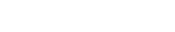This gives us an equation for x that we could solve: when we expand the square of the binomial on the right side, we will have an x-squared term on each side, and when these cancel by subtraction, what’s left will be a simple equation for x.  Once we solve this for x, the radius, we could find the area of the circle.  We don’t need to do the calculation: it’s enough to know that we could.  This statement, alone and by itself, is sufficient.

3) Let the total number of seats be N.  From the prompt, we know 60% of the seats are occupied by members and 10% by non-member guests, 0.6N and 0.1N respectively, 0.7N in total in attendance.  This means that 30% of the seats, 0.3N, are empty.  Right away, we see that the empty seats, 0.3N, are half of the members attending, 0.6N.  Statement #2 is tautological.

Each single non-member must be the guest of a single member.  Thus, if there are 0.1N seats occupied by guests, there must be another 0.1N seats occupied by members who came with a guest.  Therefore, the number of members who did not bring a guest is 0.5N, and 60% of this would be 0.3N.  If each of these 0.3N members invited a guest, there would be an additional 0.3N guests.  If we add these 0.3N guests to all the 0.7N folks already in attendance, we would get N: in other words, we would fill 100% of the seats.  Statement #1 is also tautological.

Both statements are tautological, so no information at all has been added to the prompt, and everything is insufficient.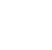If we multiply both side by b and divide both sides by c, we get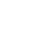Multiply both side by two; of course, anything times two is that that plus itself.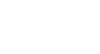Now, subtract b/d from both sides.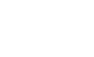This is statement #1, a direct consequence of the prompt statement.  Thus, statement #1 is a tautological statement, which adds nothing to the prompt.  The answer to the DS questions entirely depends on statement #2 alone.

Statement #2: We can take a square-root of both sides.  Normally, we would have to worry about positive/negative signs, but the prompt guarantees that all numbers are positive.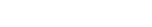Divide both sides by 5 and by d.  From the prompt, we know that the value of c/d equals the value of a/b.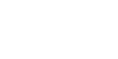Statement #2 leads directly to a numerical value for the ratio a/b.  This statement, alone and by itself, is sufficient.

•